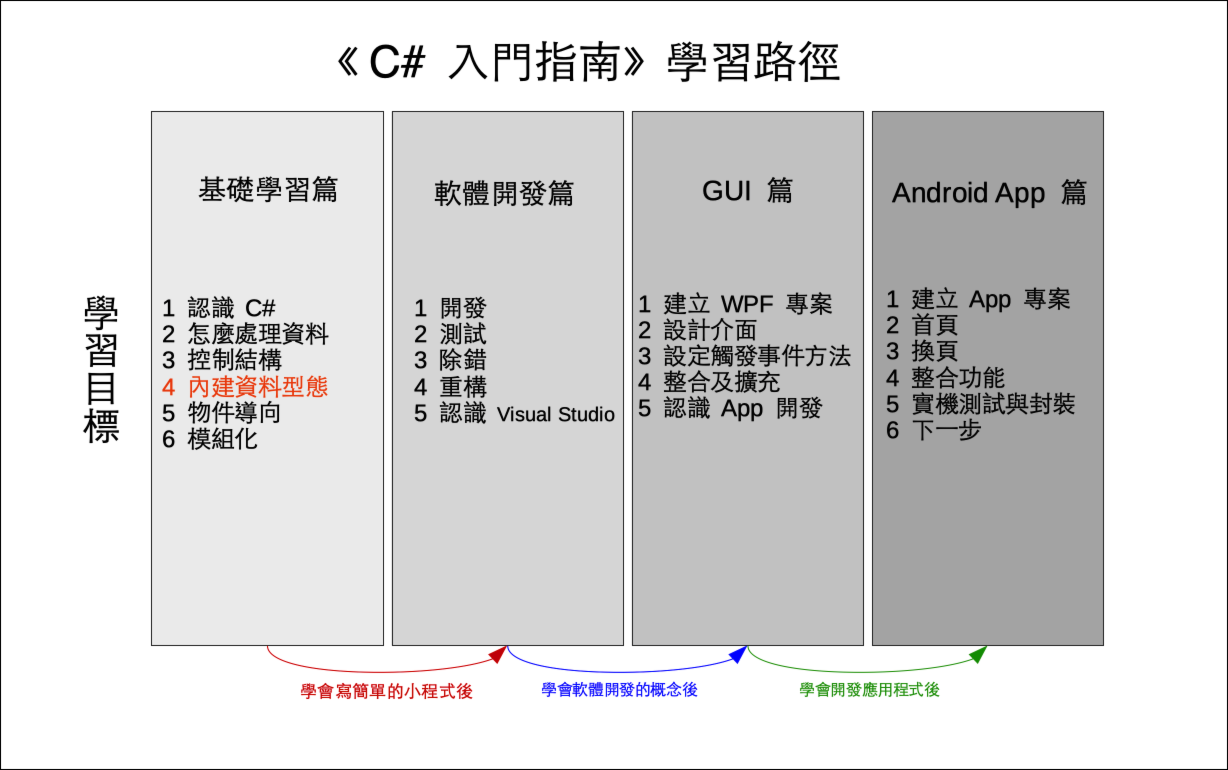# 單元 9 - 列舉與結構

～～學習進度表～～enum, struct

``enum Day {Sun, Mon, Tue, Wed, Thu, Fri, Sat};``

``````using System;

namespace EnumDemo01
{
class Program
{
enum Day {Sun, Mon, Tue, Wed, Thu, Fri, Sat};

static void Main(string[] args)
{
int a = (int) Day.Sun;
int b = (int) Day.Thu;
Console.WriteLine("Sunday: {0}", a);
Console.WriteLine("Thursday: {0}", b);
}
}
}

//《程式語言教學誌》的範例程式
// http://kaiching.org/
// 專案：EnumDemo01
// 檔名：Program.cs
// 功能：示範 C# 的列舉
// 作者：張凱慶``````

``````int a = (int) Day.Sun;
int b = (int) Day.Thu;
Console.WriteLine("Sunday: {0}", a);
Console.WriteLine("Thursday: {0}", b);``````

 C:\EnumDemo01> dotnet run Sunday: 0 Thursday: 4 C:\EnumDemo01>

``enum Range: long {Max=2000000000L, Min=1000000000L};``

``````using System;

namespace EnumDemo02
{
class Program
{
enum Range: long {Max=2000000000L, Min=1000000000L};

static void Main(string[] args)
{
long a = (long) Range.Max;
long b = (long) Range.Min;
Console.WriteLine("Max: {0}", a);
Console.WriteLine("Min: {0}", b);
}
}
}

//《程式語言教學誌》的範例程式
// http://kaiching.org/
// 專案：EnumDemo02
// 檔名：Program.cs
// 功能：示範 C# 的列舉
// 作者：張凱慶``````

 C:\EnumDemo02> dotnet run Max: 2000000000 Min: 1000000000 C:\EnumDemo02>

``````using System;

namespace StructDemo01
{
public struct Point1
{
public int x, y;
}

public struct Point2
{
public int x, y;

public Point2(int p1, int p2)
{
x = p1;
y = p2;
}

public void PrintCoordinate()
{
Console.WriteLine("({0}, {1})", x, y);
}
}

class Program
{
static void Main(string[] args)
{
Point1 p1;
p1.x = 10;
p1.y = 2;
Console.WriteLine("p1: ({0}, {1})", p1.x, p1.y);

Point2 p2 = new Point2(11, 22);
p2.PrintCoordinate();
Console.WriteLine("p2: ({0}, {1})", p2.x, p2.y);
}
}
}

//《程式語言教學誌》的範例程式
// http://kaiching.org/
// 專案：StructDemo01
// 檔名：Program.cs
// 功能：示範 C# 的結構
// 作者：張凱慶``````

``````public struct Point1
{
public int x, y;
}``````

``````Point1 p1;
p1.x = 10;
p1.y = 2;
Console.WriteLine("p1: ({0}, {1})", p1.x, p1.y);``````

``````public struct Point2
{
public int x, y;

public Point2(int p1, int p2)
{
x = p1;
y = p2;
}

public void PrintCoordinate()
{
Console.WriteLine("({0}, {1})", x, y);
}
}``````

``````public Point2(int p1, int p2)
{
x = p1;
y = p2;
}``````

「建構子」在 C# 官方文件由機器翻譯成「建構函式」，「建構函式」是沿用 C++ 的翻譯詞彙，問題是 C# 直接定義類別，並無函式（或稱函數，原文皆為 function ）的定義，因此本書採用物件導向程式設計常用的翻譯詞「建構子」。

PrintCoordinate() 沒有回傳值，宣告為 void ，參數列留空

``````public void PrintCoordinate()
{
Console.WriteLine("({0}, {1})", x, y);
}``````

``````Point2 p2 = new Point2(11, 22);
p2.PrintCoordinate();
Console.WriteLine("p2: ({0}, {1})", p2.x, p2.y);``````

 C:\StructDemo01> dotnet run p1: (10, 2) (11, 22) p2: (11, 22) C:\StructDemo01>1. C# 語言教學課程 | Microsoft Docs - 列舉
2. C# 語言教學課程 | Microsoft Docs - 結構
3. C# 參考 | Microsoft Docs - enum
4. C# 參考 | Microsoft Docs - struct
5. C# 程式設計手冊 | Microsoft Docs - 使用結構

1. 列舉是一組用識別字定義的整數常數，實際使用需要做強制型態轉換。
2. 結構則是簡單的類別，同樣可以定義欄位及方法。

1. 想一想，列舉的好處在哪裡？可以用在什麼地方？
2. 結構跟類別有不同？

1. 建立一個新專案 Exercise0901 ，利用列舉及 DateTime 物件的 DayOfWeek 屬性判斷今天是星期幾？
2. 建立一個新專案 Exercise0902 ，寫一個結構記錄商品名稱、售價、成本，然後印在命令列上。